# Work with Exact Values Involving Pi

In this worksheet, students will learn to calculate the area and circumference of circles, giving exact answers in terms of pi, in case they appear on a non-calculator paper.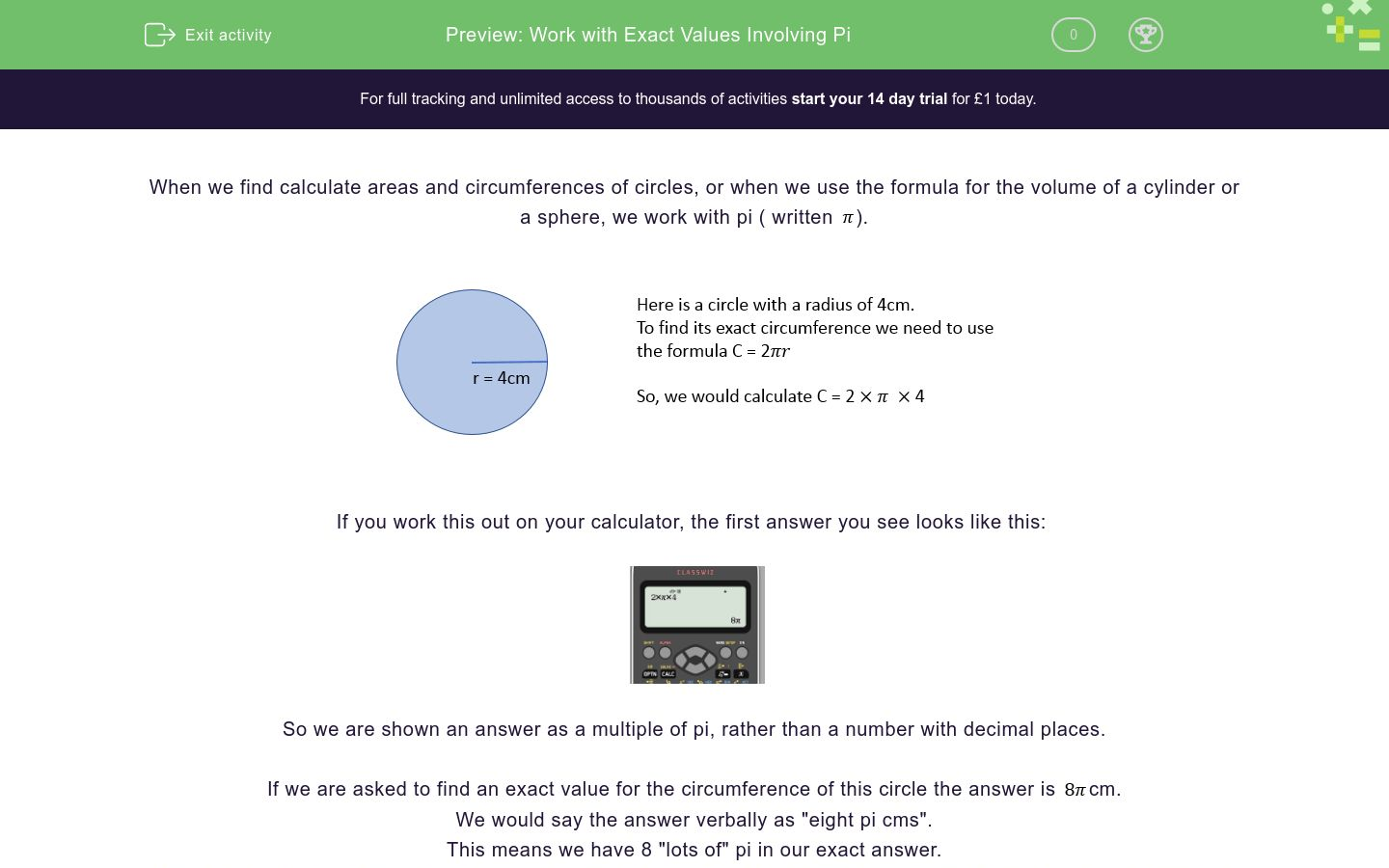Key stage:  KS 4

GCSE Subjects:   Maths

GCSE Boards:   Pearson Edexcel, OCR, Eduqas, AQA

Curriculum topic:   Number, Indices and Surds

Curriculum subtopic:   Structure and Calculation, Exact Calculations

Difficulty level:### QUESTION 1 of 10

When we find calculate areas and circumferences of circles, or when we use the formula for the volume of a cylinder or a sphere, we work with pi ( written).If you work this out on your calculator, the first answer you see looks like this:So we are shown an answer as a multiple of pi, rather than a number with decimal places.

If we are asked to find an exact value for the circumference of this circle the answer is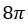cm.

We would say the answer verbally as "eight pi cms".

This means we have 8 "lots of" pi in our exact answer.

Notice that as we are expressing this using algebra, we don't use the multiplication sign (to avoid confusing it with the letter).

If we need to find the exact area of this circle we would calculate the following:The calculator gives us this answer (which is an exact answer) as a multiple of pi too:We would say that the exact area of this circle is "sixteen pi cm squared".

We can also work out these calculations mentally by multiplying (or squaring) the numbers involved in the calculations and then using this result as our multiple of pi (with correct units):Let's try some questions now to put these formulae into practise.

Try to work out the answers without a calculator, as these types of calculations can be asked on a non-calculator exam, which will mean expressing your answer in terms of &pi;.

Work out the exact circumference of this circle without a calculator:Which option shows the correct answer?

10π cm

25π cm

31.4159 cm

10 cm

Find the exact area of this circle, give your answer in terms of pi:Which option shows the correct answer?

10π cm2

25π cm2

78.5 cm2

25 cm2

Sometimes the calculations needed will include lengths or areas that are not multiples of pi.

If we are asked to find the perimeter of this shape we will need to add together the straight and curved lengths:So we need to calculate the length of the 2 straight sides, plus the circumferences of 2 semi-circles to add to this:

2 straight sides: 2 x 10 = 20 cm

2 semi-circle circumferences = the same as a whole circle circumference = 2πr = 2 x π x 3 = 6π cm

Then we need to add these together: 20 cm + 6π cm

As one term is multiplied by π but the other isn't, the simplest form of this sum is: 20 + 6π

What is the perimeter of the shape below?36 cm

43 cm

8π + 18 cm

9π + 8 cm

Let's find the area of the shape from Question 3 now.To find the area of this compound shape we need to find the area of a rectangle, plus 2 semi-circles:

Area of rectangle: 9 x 8 (same as twice the diameter) = 72 cm2

Area of 2 semi-circles = the same as a whole circle = πr2 = π x 42 = 16π cm2

Total area: 16π + 72 cm2

Now find the exact area of this shape, giving your answer in terms of pi:30 cm2

9π cm2

9π + 60 cm2

6π + 20 cm2

Questions can also focus on volume.

Remember that we find the volume of a cylinder by multiplying its cross-section by its length:Put this into practise yourself now.

Find the volume of the shape below.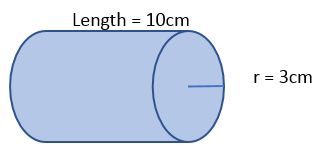30 cm2

9π cm2

9π + 60 cm2

6π + 20 cm2

We can also be asked to find the volume of a sphere

To find this, we use the formula below: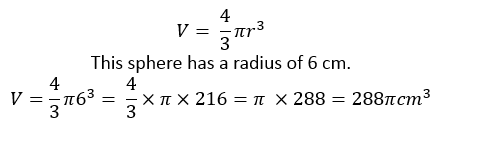So, if the radius of a sphere is 9 cm, what is its volume?

30 cm2

9π cm2

9π + 60 cm2

6π + 20 cm2

Find the exact area of the shape below, giving your answer as a multiple of pi.40π cm2

32 + 8π cm2

57 cm2

24 + 8π cm2

Find the volume of this hemisphere: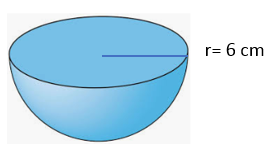40π cm2

32 + 8π cm2

57 cm2

24 + 8π cm2

Find the exact volume of this semi-cylinder.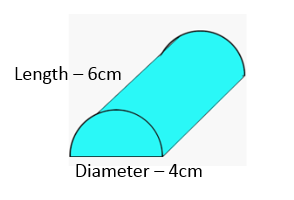40π cm2

32 + 8π cm2

57 cm2

24 + 8π cm2

This challenging question is similar to a question you might find on a Higher GCSE paper.

Here is a quarter sphere:It has a volume of 243 cm3.

We need to find the surface area of this shape.

So let's find the radius of the shape together, like this:Now is your change to give this a try yourself!

Find the curved surface area of the shape above using the formula for surface area of a sphere:40π cm2

32 + 8π cm2

57 cm2

24 + 8π cm2

• Question 1

Work out the exact circumference of this circle without a calculator:Which option shows the correct answer?

10π cm
EDDIE SAYS
What formula do you use to calculate the circumference of a circle? C = 2πr Let's put the radius (5) in the place of 'r': C = 2 x π x 5 = 10π As the question asks you not to use a calculator and uses the word exact, this tells you an answer in terms of π will be much more accurate than a decimal. How was that one?
• Question 2

Find the exact area of this circle, give your answer in terms of pi:Which option shows the correct answer?

25π cm2
EDDIE SAYS
What is the formula to calculate the area of a circle? A = πr2 Let's substitute the radius in the circle above (5) for the letter 'r' in this equation: A = π x 52 = 25π Don't forget that unit! And we need to square it as it represents an area, rather than a straight line distance. In this case, it's cm2.
• Question 3

Sometimes the calculations needed will include lengths or areas that are not multiples of pi.

If we are asked to find the perimeter of this shape we will need to add together the straight and curved lengths:So we need to calculate the length of the 2 straight sides, plus the circumferences of 2 semi-circles to add to this:

2 straight sides: 2 x 10 = 20 cm

2 semi-circle circumferences = the same as a whole circle circumference = 2πr = 2 x π x 3 = 6π cm

Then we need to add these together: 20 cm + 6π cm

As one term is multiplied by π but the other isn't, the simplest form of this sum is: 20 + 6π

What is the perimeter of the shape below?9π + 8 cm
EDDIE SAYS
Did you add your straight sides and semi-circles separately? 2 straight sides: 2 x 9 = 18 cm 2 semi-circle circumferences: 2πr = 2 x π x 4 = 8π cm If we add these together, we reach: 18 + 8π cm As π is not a factor of both numbers, we cannot simplify this expression any further.
• Question 4

Let's find the area of the shape from Question 3 now.To find the area of this compound shape we need to find the area of a rectangle, plus 2 semi-circles:

Area of rectangle: 9 x 8 (same as twice the diameter) = 72 cm2

Area of 2 semi-circles = the same as a whole circle = πr2 = π x 42 = 16π cm2

Total area: 16π + 72 cm2

Now find the exact area of this shape, giving your answer in terms of pi:9π + 60 cm2
EDDIE SAYS
Area of rectangle: 10 x 6 (same as twice the diameter) = 60 cm2 Area of 2 semi-circles = the same as a whole circle = πr2 = π x 32 = 9π cm2 Total area: 9π + 60 cm2
• Question 5

Questions can also focus on volume.

Remember that we find the volume of a cylinder by multiplying its cross-section by its length:Put this into practise yourself now.

Find the volume of the shape below.EDDIE SAYS
How do you find the volume of a cylinder? V = πr2h Here the radius ('r') is 3 cm and the length ('h') is 10 cm. If we substitute these into the equation, we reach: V = π x 32 x 10 = 90π cm3
• Question 6

We can also be asked to find the volume of a sphere

To find this, we use the formula below:So, if the radius of a sphere is 9 cm, what is its volume?

EDDIE SAYS
To find the volume of a sphere, we need to use the formula: V = 4/3 πr3 The sphere has a radius ('r') of 9, so let's substitute this in the equation: V = 4/3 x π x 93 = 4/3 x π x 729 = 972π cm3
• Question 7

Find the exact area of the shape below, giving your answer as a multiple of pi.32 + 8π cm2
EDDIE SAYS
Area of rectangle: 4 x 8 = 32 cm2 Area of one semi-circle: 1/2 x (π x 42) = 1/2 x 16π = 8π cm2 Total of two: 8π + 32 cm2
• Question 8

Find the volume of this hemisphere:EDDIE SAYS
Here we need to find the volume of a whole sphere and then halve it. What formula do we use to find the volume of a sphere? V = 4/3πr3 Let's substitute 6 for 'r' here: V = 4/3 x π x 63 = 4/3 x π x 216 = 288π Now let's halve this, as we only see half of the sphere present: 1/2 x 288π = 144π cm3
• Question 9

Find the exact volume of this semi-cylinder.EDDIE SAYS
Here we need to find half the volume of the whole cylinder. How do we find the volume of a cylinder?• Question 10

This challenging question is similar to a question you might find on a Higher GCSE paper.

Here is a quarter sphere:It has a volume of 243 cm3.

We need to find the surface area of this shape.

So let's find the radius of the shape together, like this:Now is your change to give this a try yourself!

Find the curved surface area of the shape above using the formula for surface area of a sphere:EDDIE SAYS
The surface area of the curved element can be found by applying the formula given: SA = (4πr2) ÷ 4 We know that the value of 'r' is 9 so let's add that in: SA = (4 x π x 92) ÷ 4 SA = 324π ÷ 4 = 81π cm3 To find the surface area of the 2 semi-circles (which is the same as one full circle), we need to the formula for the area of a circle: A = πr2 Let's add 9 in to represent 'r' again: A = π x 92 = 81π Curved surface area: 81π cm3 Surface area of 2 semi-circles: 81π cm3 If we add these together, we reach an overall answer of: 162π cm3 Well done for working hard on these tough circle questions! Why not practise working on circles some more if you found these tricky?
---- OR ----

Sign up for a £1 trial so you can track and measure your child's progress on this activity.

### What is EdPlace?

We're your National Curriculum aligned online education content provider helping each child succeed in English, maths and science from year 1 to GCSE. With an EdPlace account you’ll be able to track and measure progress, helping each child achieve their best. We build confidence and attainment by personalising each child’s learning at a level that suits them.

Get started